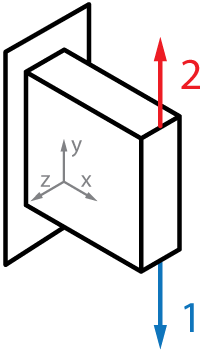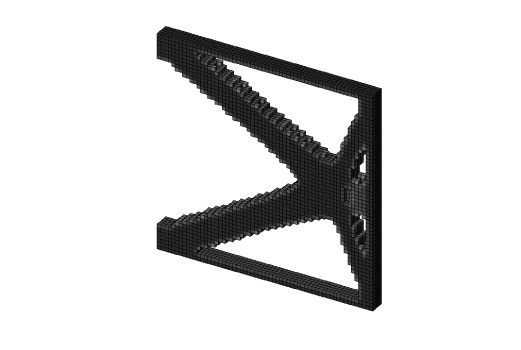# Multiple load cases - Top3d

In this tutorial, you will learn how to modify the original 3d topology optimization program (top3d) to solve multiple load cases problem.

In order to solve a multiple load cases problem, as shown below, a few changes need to be made.First, the loading conditions (line 12) are changed correspondingly:

il = [nelx nelx]; jl = [0 nely]; kl = [nelz/2 nelz/2];


Also the force vector (line 22) and displacement vector (line 23) become more than one column:

F = sparse(loaddof,[1 2],[-1 1],ndof,2);
U = zeros(ndof,2);


# Step.2: Define Objective Functions

The objective function is now the sum of different load cases

where $M$ is the number of load cases.

Then lines 74-76 are substituted with lines

c = 0.;
dc = zeros(nely,nelx,nelz);
for i = 1:size(F,2)
Ue = U(:,i);
ce = reshape(sum((Ue(edofMat)*KE).*Ue(edofMat),2),[nely,nelx,nelz]);
c = c + sum(sum(sum((Emin+xPhys.^penal*(E0-Emin)).*ce)));
dc = dc - penal*(E0-Emin)*xPhys.^(penal-1).*ce;
end


# Step.3: Run program

This example is promoted by the line

top3d(60,60,4,0.4,3.0,1.5)


You will have similar result like this